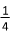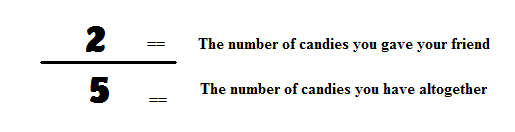# What Are Fractions?

A fraction is a number that represents a whole number that has been divided into equal parts. For example, if you have a pie and you cut it into 4 equal slices, 1 of those slices is written as, as shown here:The shaded area represents 1 of the 4 slices of the pie. It is written as. A fraction can also represent a part of a group. For example, if you have 5 pieces of candy and you give 2 to your friend, those 2 candies are written as, as shown here:The shaded area represents 2 of the 5 candies. It is written as.

A fraction is made up of two parts, the denominator and the numerator.

The denominator is the bottom number of the fraction. It is the number that represents the whole amount.

The numerator is the top number of the fraction. It is the number that represents the part of the whole.